# Car Number Plate Detection Using MATLAB and Image Processing

Published  November 26, 2018   16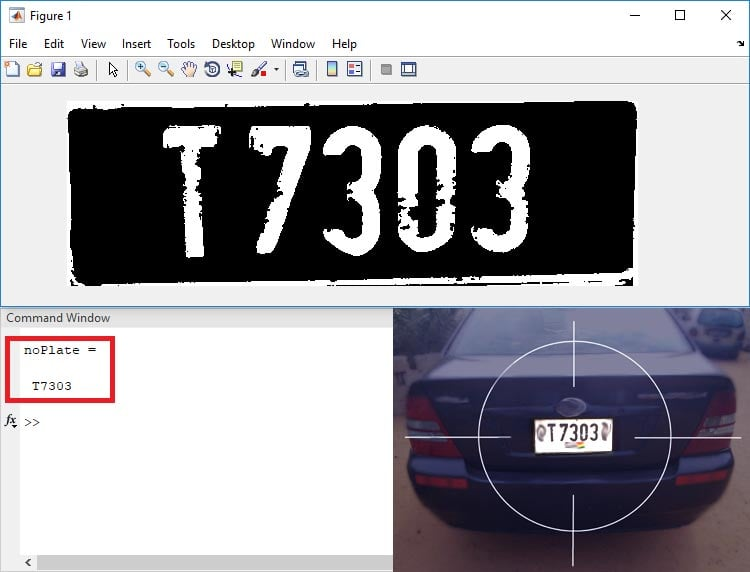Have you ever wonder that how an ANPR (Automatic Number Plate Recognition) system works? Let me tell you the concept behind it, the camera of the ANPR system captures image of vehicle license plate and then the image is processed through multiple number of algorithms to provide an alpha numeric conversion of the image into a text format. ANPR system is used at many places like Petrol Pumps, Shopping Malls, Airports, highways, toll booths, Hotels, Hospitals, Parking lots, Defense & Military check points etc.

There are many image processing tools available for this Number plate detection, but here in this tutorial we will use MATLAB Image Processing to get the vehicle license plate number into the text format. If you are new with MATLAB or image processing, then check our previous MATLAB projects:

First, let me brief you about the concept we are using for detecting number plates. There are three programs or ‘.m’ files for this project.

• Template Creation (template_creation.m)– This is used to call the saved images of alphanumerics and then save them as a new template in MATLAB memory.
• Letter Detection(Letter_detection.m) – Reads the characters from the input image and find the highest matched corresponding alphanumeric.
• Plate Detection(Plate_detection.m) – Process the image and then call the above two m-files to detect the number.

Now, we will learn about how to code these m-files and what you have to do before start coding. After going through this tutorial, you can find all the code files and working explanation video at the end of this project.

### Template Creation

First create a folder for the project (my folder name is Number Plate Detection) to save and store the files. We have stored the binary images of all the alphabets and numbers in the sub-folder named as ‘alpha'.

Now, open the Editor window in the MATLAB, as shown in the below image,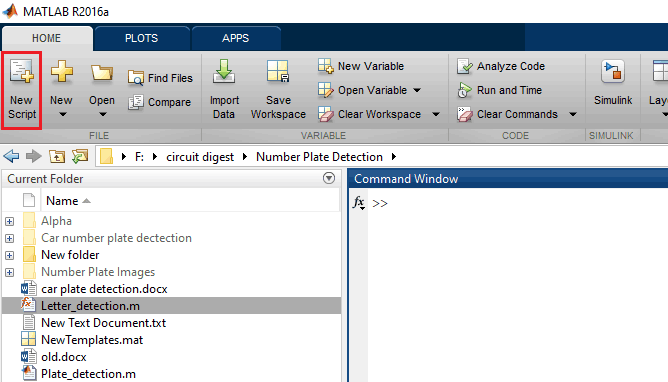If you are not familiar with the basic terminology of MATLAB I suggest you to check the linked tutorial.

Now, copy and paste the below code in template_creation.m file, and save the file in the project folder (Number Plate Detection). All the files related to this project including image templates files can be downloaded from here. Also check the video given at the end of this project.

```%Alphabets

%Natural Numbers

%Creating Array for Alphabets
letter=[A B C D E F G H I J K L M N O P Q R S T U V W X Y Z];

%Creating Array for Numbers
number=[one two three four five six seven eight nine zero];

NewTemplates=[letter number];
save ('NewTemplates','NewTemplates')
clear all```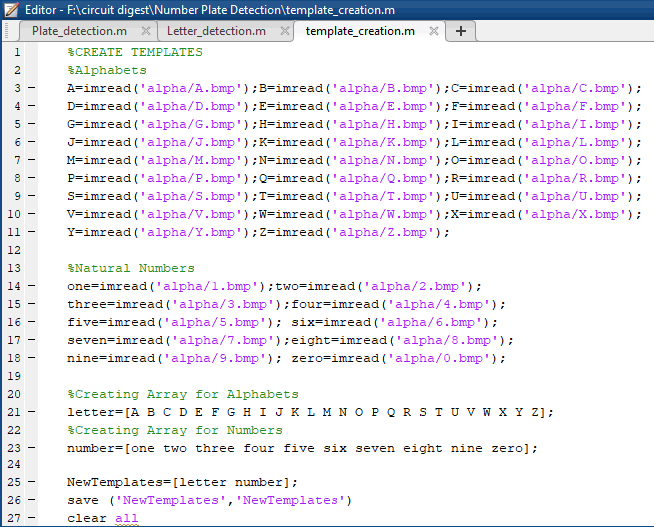Here, in the above code we are saving the images into a variable by using command imread(). This function is used to call the images from the folder or from any location of the PC into the MATLAB. Let’s take an example from the above code:

`A=imread('alpha/A.bmp');`

Where A is the variable, and in ‘alpha/A.bmp’, ‘alpha’ is the folder name and ‘A.bmp’ is the file name.

Then create a matrix of ‘letter’ and ‘number’ and save it in variable ‘NewTemplates’ by using command ‘save(filename,variables)’.

```%Creating Array for Alphabets
letter=[A B C D E F G H I J K L M N O P Q R S T U V W X Y Z];

%Creating Array for Numbers
number=[one two three four five six seven eight nine zero];

NewTemplates=[letter number];
save ('NewTemplates','NewTemplates')
clear all```

Now start coding Letter_detection.m, in a new editor window.

### Letter Detection

Here we are creating the second code file named Letter_detection.m. Now, copy and paste the below code in that file and save the file in the project folder with name Letter_detection. This file can be downloaded from here, this attached zip files also contains other files related to this Number plate detection project.

```function letter=readLetter(snap)

snap=imresize(snap,[42 24]);
rec=[ ];

for n=1:length(NewTemplates)
cor=corr2(NewTemplates{1,n},snap);
rec=[rec cor];
end

ind=find(rec==max(rec));
display(find(rec==max(rec)));

% Alphabets listings.
if ind==1 || ind==2
letter='A';
elseif ind==3 || ind==4
letter='B';
elseif ind==5
letter='C'
elseif ind==6 || ind==7
letter='D';
elseif ind==8
letter='E';
elseif ind==9
letter='F';
elseif ind==10
letter='G';
elseif ind==11
letter='H';
elseif ind==12
letter='I';
elseif ind==13
letter='J';
elseif ind==14
letter='K';
elseif ind==15
letter='L';
elseif ind==16
letter='M';
elseif ind==17
letter='N';
elseif ind==18 || ind==19
letter='O';
elseif ind==20 || ind==21
letter='P';
elseif ind==22 || ind==23
letter='Q';
elseif ind==24 || ind==25
letter='R';
elseif ind==26
letter='S';
elseif ind==27
letter='T';
elseif ind==28
letter='U';
elseif ind==29
letter='V';
elseif ind==30
letter='W';
elseif ind==31
letter='X';
elseif ind==32
letter='Y';
elseif ind==33
letter='Z';
%*-*-*-*-*
​
% Numerals listings.
elseif ind==34
letter='1';
elseif ind==35
letter='2';
elseif ind==36
letter='3';
elseif ind==37 || ind==38
letter='4';
elseif ind==39
letter='5';
elseif ind==40 || ind==41 || ind==42
letter='6';
elseif ind==43
letter='7';
elseif ind==44 || ind==45
letter='8';
elseif ind==46 || ind==47 || ind==48
letter='9';
else
letter='0';
end
end```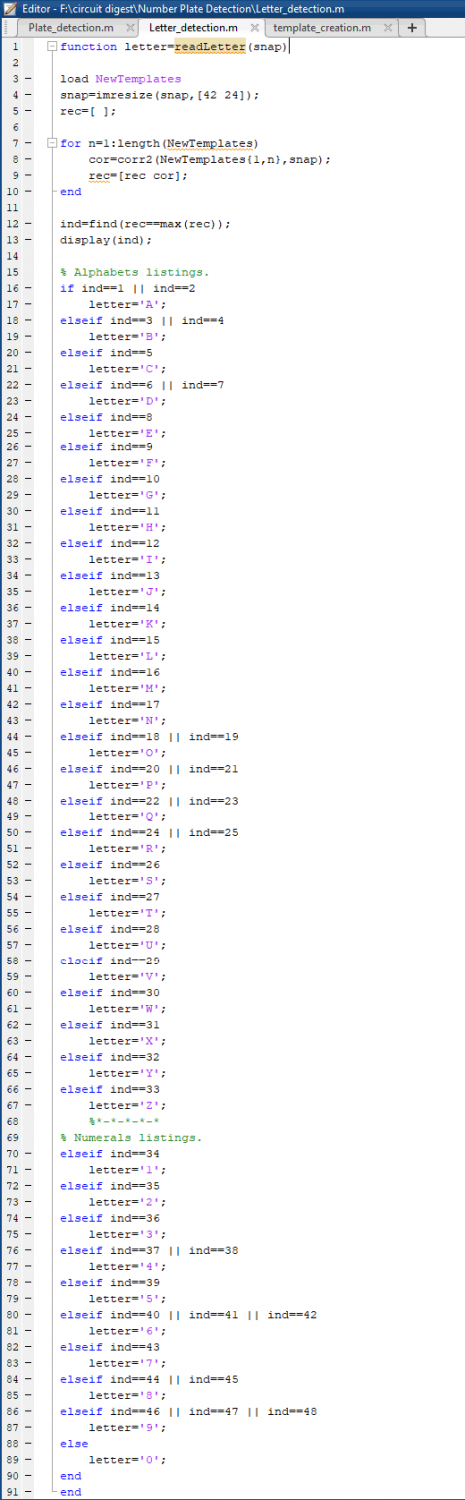Here, in the above code we have created a function named letter which gives us the alphanumeric output of the input image from class ‘alpha’ by using command ‘readLetter()’. And then load the saved templates by using command load ‘NewTemplates.

After that, we have resized the input image so it can be compared with the template’s images by using the command ‘imresize(filename,size)’. Then for loop is used to correlates the input image with every image in the template to get the best match.

A matrix ‘rec’ is created to record the value of correlation for each alphanumeric template with the characters template from the input image, as shown in the below code,

`cor=corr2(NewTemplates{1,n},snap);`

Then ‘find()’ command is used to find the index which corresponds to the highest matched character. Then according to that index, corresponding character is printed using‘if-else’ statement.

Now, after completing with this open a new editor window to start code for the main program.

### Number Plate Detection

Here is the third and final code file named Plate_detection.m copy and paste the below code in this file and save into the project folder. For quick start you can download all the code files with image templates from here.

```close all;
clear all;

im = imread(' Number Plate Images/ image1.png');
imgray = rgb2gray(im);
imbin = imbinarize(imgray);
im = edge(imgray, 'prewitt');

%Below steps are to find location of number plate
Iprops=regionprops(im,'BoundingBox','Area', 'Image');
area = Iprops.Area;
count = numel(Iprops);
maxa= area;
boundingBox = Iprops.BoundingBox;
for i=1:count
if maxa<Iprops(i).Area
maxa=Iprops(i).Area;
​ boundingBox=Iprops(i).BoundingBox;
end
end

im = imcrop(imbin, boundingBox);
im = bwareaopen(~im, 500);
[h, w] = size(im);

imshow(im);

Iprops=regionprops(im,'BoundingBox','Area', 'Image');
count = numel(Iprops);
noPlate=[];
for i=1:count
ow = length(Iprops(i).Image(1,:));
oh = length(Iprops(i).Image(:,1));
if ow<(h/2) & oh>(h/3)
letter=Letter_detection(Iprops(i).Image);
noPlate=[noPlate letter]
end
end```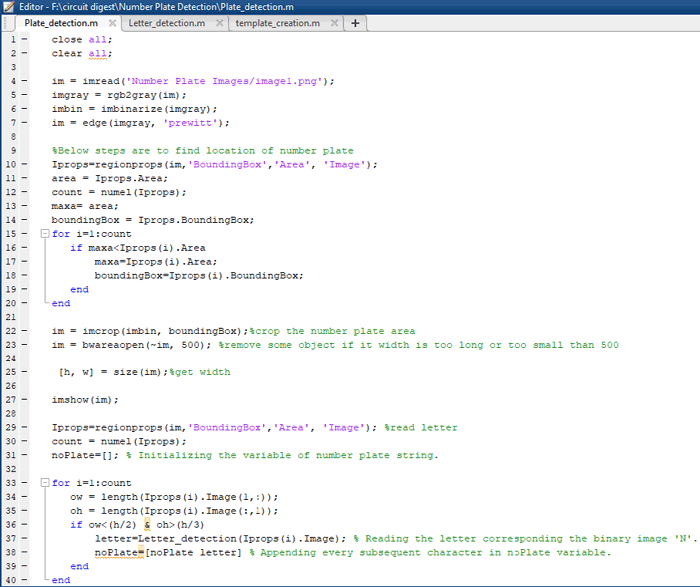Basic commands used in above code are mentioned below:

imread() – This command is used to open the image into the MATLAB from the target folder.

rgb2gray() –This command is used to convert the RGB image into grayscale format.

imbinarize() – This command is used to Binarize 2-D grayscale image or simply we can say it converts the image into black and white format.

edge() – This command is used to detect the edges in the image, by using various methods like Roberts, Sobel, Prewitt and many others.

regionprops() – This command is used to measure properties of image region.

numel() – This command is used to calculate the number of array elements.

imcrop() – This command is used to crop the image in the entered size.

bwareaopen() – This command is used to remove small objects from binary image.

By using the above commands in the code, we are calling the input image and converting it into the grayscale. Then the grayscale is converted into the binary image, and the edge of the binary images is detected by the Prewitt method.

Then the below code is used to detect the location of the number plate in the entire input image,

```Iprops=regionprops(im,'BoundingBox','Area', 'Image');
area = Iprops.Area;
count = numel(Iprops);
maxa= area;
boundingBox = Iprops.BoundingBox;
for i=1:count
if maxa<Iprops(i).Area
maxa=Iprops(i).Area;
boundingBox=Iprops(i).BoundingBox;
end
end   ```

After that crop the number plate and remove the small objects from the binary image by using command ‘imcrop()’ and ‘bwareaopen()’ respectively.

Then, the below code is used to process that cropped license plate image and to display the detected number in the image and text format (in the command window).

```Iprops=regionprops(im,'BoundingBox','Area', 'Image');
count = numel(Iprops);
noPlate=[];

for i=1:count
ow = length(Iprops(i).Image(1,:));
oh = length(Iprops(i).Image(:,1));
if ow<(h/2) & oh>(h/3)
letter=Letter_detection(Iprops(i).Image);
noPlate=[noPlate letter]
end
end```

### Working of Vehicle License Plate Number Detection System using MATLAB

In the template_creation.m file we have design the code to save all the binary images of alphanumerics into a directory or file named as ‘NewTemplates’. Then that directory is called in the Letter_detection.m as you can see in the below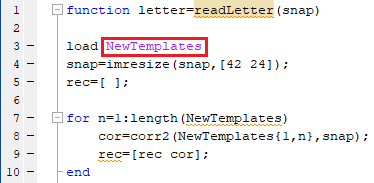Then in the Plate_detection.m code file the Letter_detection.m code file is called when we process the image as shown in image below,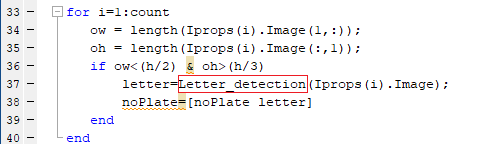Now, click on the ‘RUN’ button to run the .m file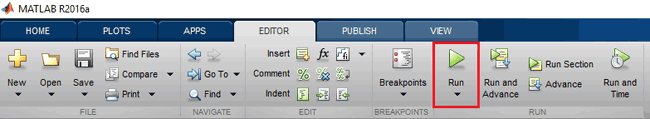MATLAB may take few seconds to respond, wait until it shows busy message in the lower left corner as shown below,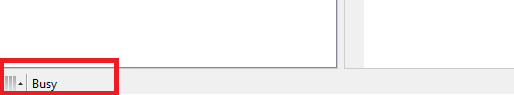As the program start you will get the number plate image popup and the number in the command window. The output for my image will look like the image given below;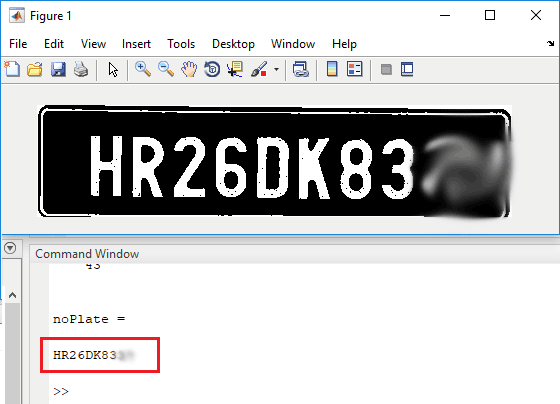Complete working of Vehicle License Number Plate Detection System is demonstrated in the Video below, and all the code files with image templates can be downloaded from here.

Also, check all MATLAB Projects here.

### showing error in letter detection

When i am running letter detection, it is showing :

Brace indexing is not supported for variables of this type.

Error in Letter_detection (line 8)
cor=corr2(NewTemplates{1,n},snap);

### Brace indexing is not

Brace indexing is not supported for variables of this type.

Error in Letter_detection (line 8)
cor=corr2(NewTemplates{1,n},snap);

### Error while running the code

Brace indexing is not supported for variables of this type.

Error in Letter_detection (line 8)
cor=corr2(NewTemplates{1,n},snap);

### error in program letter_detection.m

in the code lines

for n=1:length(NewTemplates)
cor=corr2(NewTemplates(1,n),snap);
rec=[rec cor];
end

first of all brace indexing is not supported in higher versions of matlab. Whenever I try to run the program it says that Error using corr2>ParseInputs (line 39)
A and B must be the same size.

I need help with that.

### That's Because you Run The

That's Because you Run The Wrong File And So Theresn't Any Picture To Calculate the corr2. Follow the Below Guides

The imbinarize function in Plate Detection.m File deosn't work on matlab 2015 or the other lower vesions.

and also Remember that you must run the Plate Detection.m file.

Not the Letter Detection.m File

if The Problem Doesn't Solve Check My GitHub Repo :

https://github.com/hamidKMB/Car-Number-Plate-Detection/blob/main/vehicle-number-plate-detection-Final/Plate_detection.m

### Sir I got the following

Sir I got the following errors while running the plate detection file. Plz help to solve it.

Brace indexing is not supported for variables of this type.

cor=corr2(NewTemplates{1,n},snap);

letter=Letter_detection(Iprops(i).Image); % Reading the letter corresponding the binary image
'N'.

The imbinarize function in Plate Detection.m File deosn't work on matlab 2015 or the other lower vesions.

and also Remember that you must run the Plate Detection.m file.

Not the Letter Detection.m File

### To All People Who Stuck On

To All People Who Stuck On this Code.

So first of All Remember That You Must Run the Plate Detection.m File.
The second Advice is that the imbinarize() function Doesn't work on MATLAB 2015 And Other Lower Versions.

If all of the Above Guides Doesn't Help you So Checkout My GitHub Repo.
I Made some Changes On the Code So it Works Fine.

!!!!!!!!!  REMEMBER THAT YOU MUST RUN THE Plate Detection.m FILE !!!!!!!!!!!!!!

https://github.com/hamidKMB/Car-Number-Plate-Detection/blob/main/vehicle-number-plate-detection-Final/Plate_detection.m

### rec=[ ];

```rec=[ ];

for n=1:length(NewTemplates)
cor=corr2(NewTemplates{1,n},snap);
rec=[rec cor];
end```

```if ind==1 || ind==2
letter='A';
elseif ind==3 || ind==4
letter='B';```

Can someone please explain these two portions?

And why we are having two find values for some alphabets and letters??